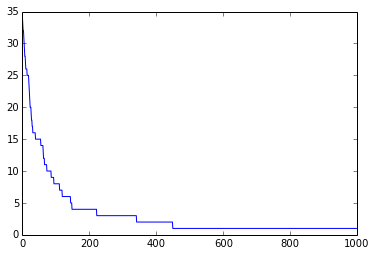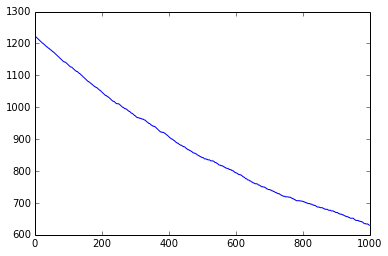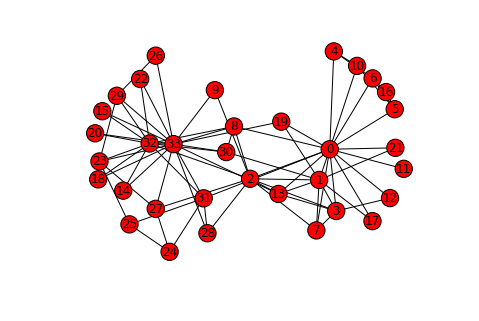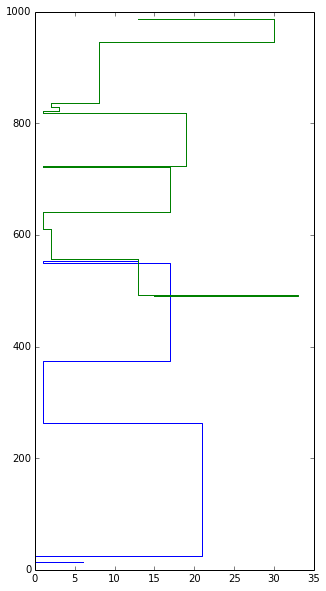# Social Learning¶

## The Voter Model¶

This model of social learning was independently introduced in Clifford and Sudbury (1973), Holley and Liggett (1975). The setting and dynamic process are the following:

• Each agent has its own opinion
• On each iteration a randoly choosen agent chooses its neighbour and adopts its side

It was shown that agents' states reach a consensus over any connected graph $G$ (Coalescing Random Walks).

In :
import numpy as np
import scipy as sp
import networkx as nx
import matplotlib.pyplot as plt
%matplotlib inline


Implement the Voter Model and check how the number of agents' states changes with time. Run it for Karate club and Political Blogs networks.

In :
G = nx.karate_club_graph()
y = range(G.number_of_nodes())

maxIter = 1000
numStates = np.zeros((maxIter,), dtype=int)
path = []

for i in xrange(maxIter):
numStates[i] = len(np.unique(y))
agent = np.random.choice(G.nodes())


In :
plt.plot(numStates)

Out:
[<matplotlib.lines.Line2D at 0x7f439625f050>]In :
numStates[-1]

Out:
1
In :
# Some preprocessing for polblogs
G = nx.Graph(A)
for n,d in G.degree_iter():
if d == 0:
G.remove_node(n)

mapping = {}
i = 0
for n in G.nodes_iter():
mapping[n] = i
i+=1
G = nx.relabel_nodes(G,mapping)

In :
plt.plot(numStates)

Out:
[<matplotlib.lines.Line2D at 0x7f4361143710>]Try to write a code that identifies the path of opinion adoption starting from a particular node

In :
G = nx.karate_club_graph()
y = range(G.number_of_nodes())

maxIter = 1000
numStates = np.zeros((maxIter,), dtype=int)
path = []

for i in xrange(maxIter):
numStates[i] = len(np.unique(y))
agent = np.random.choice(G.nodes())

In :
path[0:3]

Out:
[(16, 5), (3, 12), (2, 0)]
In :
nx.draw(G, with_labels=True)In [ ]:


In :
start = 6

rw = []
time = []
t = 0

for hop in path:
if hop == start:
rw.append(hop)
time.append(t)
start = hop
t+=1

In :
pltrw = []
plttime = []

for i in xrange(len(rw)-1):
pltrw.extend((rw[i], rw[i], rw[i]))
plttime.extend((time[i], time[i], time[i+1]))

pltrw.extend((rw[-1], rw))
plttime.extend((time[-1], time[-1]))

In :
pltrw2 = pltrw[:]
plttime2 = plttime[:]

In :
plt.figure(figsize=(5,10))
plt.plot(pltrw, plttime, pltrw2, plttime2)

Out:
[<matplotlib.lines.Line2D at 0x7f4360d83710>,
<matplotlib.lines.Line2D at 0x7f4360d83990>]1. Clifford, P., and A. Sudbury, "A model for spatial conflict", 1973.
2. Holley R and Liggett T M, "Ergodic Theorems for Weakly Interacting Infinite Systems and the Voter Model", 1975.
3. Ercan Yildiz , Daron Acemoglu , Asuman Ozdaglar , Amin Saberi , Anna Scaglione, "Discrete Opinion Dynamics with Stubborn Agents", 2011
4. Abhimanyu Das, Sreenivas Gollapudi, and Kamesh Munagala, "Modeling Opinion Dynamics in Social Networks", 2014